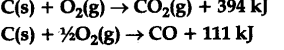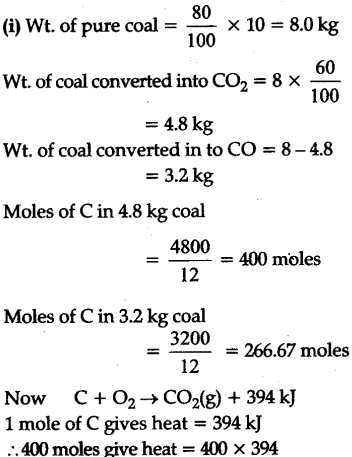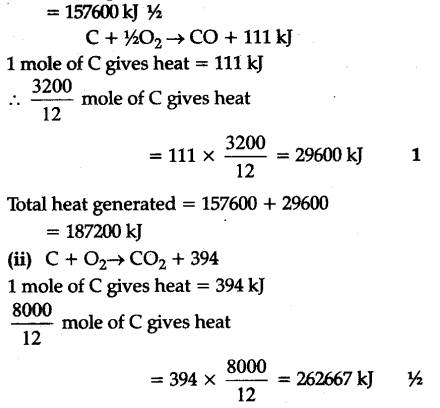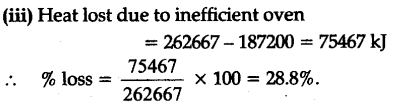# Find out the values(i) In an oven using coal (assume the coal is carbon in weight), insufficient oxygen is sup-plied such that 60% of carbon is converted to C\${{O}{2}} and 40% carbon is converted to CO. Find out the heat generated when 10 kg of coal is burnt in this fashion. (ii) Calculate the heat generated if a more efficient oven is used so that only C{{O}{2}}\$ is formed.
(iii) Calculate the percentage loss in heating value for the inefficient oven.